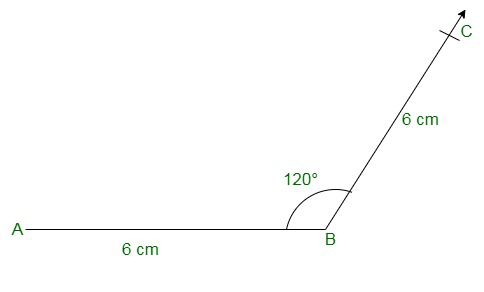Open in App
Not now

# Class 8 RD Sharma Solutions – Chapter 18 Practical Geometry – Exercise 18.3

• Last Updated : 05 May, 2021

### Question 1: Construct a quadrilateral ABCD in which AB = 3.8 cm, BC = 3.4 cm, CD = 4.5 cm, AD = 5 cm and ∠B = 80°?

Solution:

The given details are AB = 3.8 cm, BC = 3.4 cm, CD = 4.5 cm, AD = 5 cm and ∠B = 80°.

Step 1: Draw a line segment AB = 3.8cm

Step 2: Construct an angle of 80o at B.

Step 3: Taking B as the centre, draw an arc radius of 3.4 cm. Mark this point as C.

Step 4: Taking A as the centre, draw an arc radius of 5 cm. Mark this point as D.

Step 5: Taking C as the centre, draw an arc radius of 4.5 cm intersecting point D.

Step 6: Now join BC, AD, and CD to form a quadrilateral.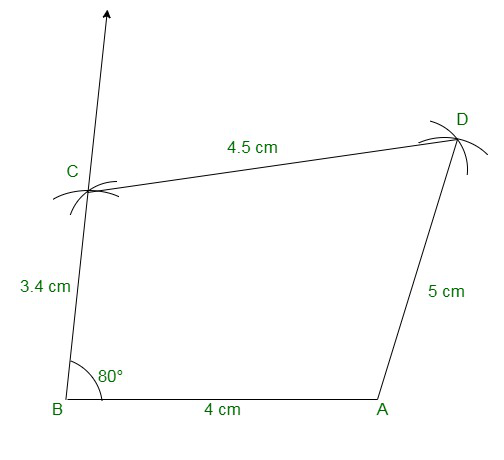### Question 2: Construct a quadrilateral ABCD given that AB = 8 cm, BC = 8 cm, CD = 10 cm, AD = 10 cm and ∠A = 45°?

Solution:

The given details are AB = 8 cm, BC = 8 cm, CD = 10 cm, AD = 10 cm and ∠A = 45°.

Step 1: Draw a line segment AB = 8cm

Step 2: Construct an angle of 45o at A.

Step 3: Taking A as the centre, draw an arc radius of 10 cm. Mark this point as D.

Step 4: Taking D as the centre, draw an arc radius of 10 cm. Mark this point as C.

Step 5: Taking B as the centre, draw an arc radius 8 cm intersecting point C.

Step 6: Now join AD, DC, and BC to form a quadrilateral.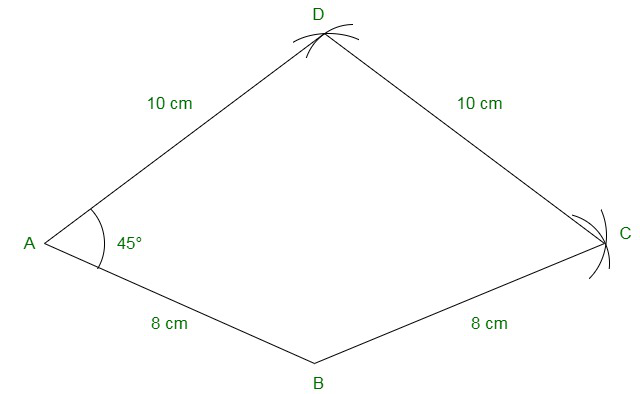### Question 3: Construct a quadrilateral ABCD in which AB = 7.7 cm, BC = 6.8 cm, CD = 5.1 cm, AS = 3.6 cm and ∠C = 120°?

Solution:

We have, AB = 7.7 cm, BC = 6.8 cm, CD = 5.1 cm, AS = 3.6 cm and ∠C = 120°.

Step 1: Draw a line segment , DC = 5.1cm

Step 2: Construct an angle of 120° at C.

Step 3: Taking C as the centre, draw an arc radius of 6.8 cm. Mark this point as B.

Step 4: Taking B as the centre, draw an arc radius of 7.7 cm. Mark this point as A.

Step 5: Taking D as the centre, draw an arc radius of 3.6 cm intersecting point A.

Step 6: Now join CB, BA, and DA to form a quadrilateral.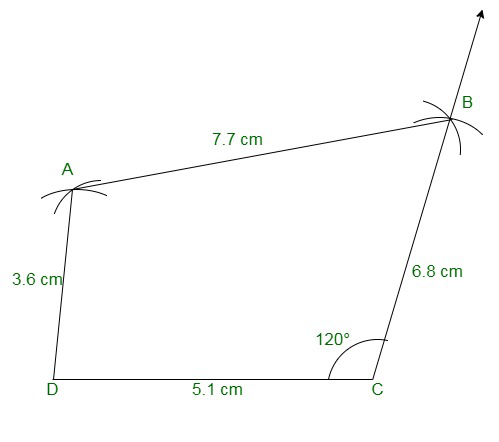### Question 4: Construct a quadrilateral ABCD in which AB = BC = 3 cm, AD = CD = 5 cm and ∠B = 120°?

Solution:

We have, AB = BC = 3 cm, AD = CD = 5 cm and ∠B = 120°.

Step 1: Draw a line segment AB = 3cm

Step 2: Construct an angle of 120° at B.

Step 3: Taking B as the centre, draw an arc radius of 3 cm. Mark this point as C.

Step 4: Taking C as the centre, draw an arc radius of 5 cm. Mark this point as D.

Step 5: Taking A as the centre, draw an arc radius of 5 cm intersecting point D.

Step 6: Now join BC, CD, and DA to form a quadrilateral.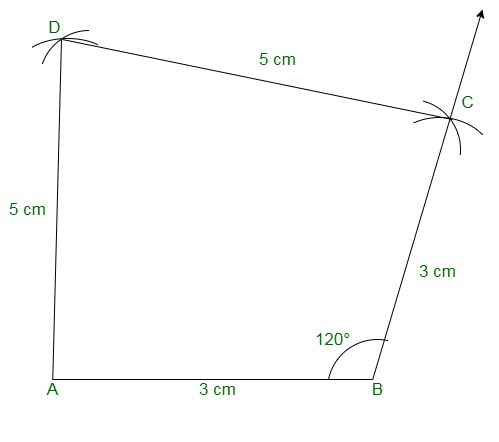### Question 5: Construct a quadrilateral ABCD in which AB = 2.8 cm, BC = 3.1 cm, CD = 2.6 cm and DA = 3.3 cm and ∠A = 60°?

Solution:

We have, AB = 2.8 cm, BC = 3.1 cm, CD = 2.6 cm and DA = 3.3 cm and ∠A = 60°.

Step 1: Draw a line segment AB = 2.8cm

Step 2: Construct an angle of 60° at A.

Step 3: Taking A as the centre, draw an arc radius of 5.5 cm. Mark this point as D.

Step 4: Taking D as the centre, draw an arc radius of 2.6 cm. Mark this point as C.

Step 5: Taking B as the centre, draw an arc radius of 3.1 cm. Mark this point as C.

Step 6: Now join AD, DC, and CB to form a quadrilateral.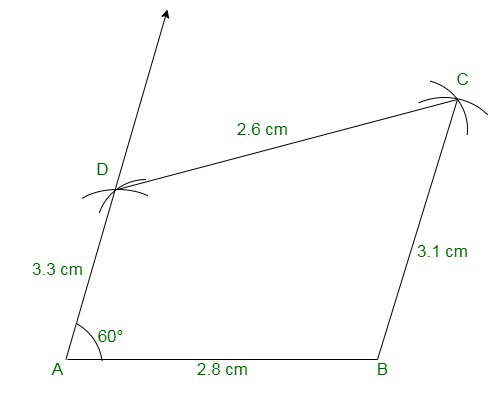### Question 6: Construct a quadrilateral ABCD in which AB = BC = 6 cm, AD = DC = 4.5 cm and ∠B = 120°?

Solution:

We have, AB = BC = 6 cm, AD = DC = 4.5 cm and ∠B = 120°.

Step 1: Draw a line segment AB = 6cm

Step 2: Construct an angle of 120° at B.

Step 3: Taking B as the centre, draw an arc radius of 6 cm. Mark this point as C.

Here, AC is about 10.3 cm in length which is greater than AD + CD = 4.5 + 4.5 = 9cm

We know that sum of the two sides of a triangle is always greater than the third side.